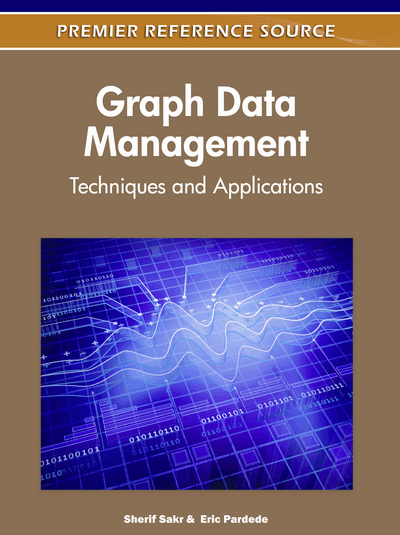# Efficient Techniques for Graph Searching and Biological Network Mining

Alfredo Ferro (Università di Catania, Italy), Rosalba Giugno (Università di Catania, Italy), Alfredo Pulvirenti (Università di Catania, Italy) and Dennis Shasha (Courant Institute of Mathematical Sciences, USA)
DOI: 10.4018/978-1-61350-053-8.ch005
Available
\$37.50
No Current Special Offers

## Abstract

From biochemical applications to social networks, graphs represent data. Comparing graphs or searching for motifs on such data often reveals interesting and useful patterns. Most of the problems on graphs are known to be NP-complete. Because of the computational complexity of subgraph matching, reducing the candidate graphs or restricting the space in which to search for motifs is critical to achieving efficiency. Therefore, to optimize and engineer isomorphism algorithms, design indexing and suitable search methods for large graphs are the main directions investigated in the graph searching area. This chapter focuses on the key concepts underlying the existing algorithms. First it reviews the most known used algorithms to compare two algorithms and then it describes the algorithms to search on large graphs making emphasis on their application on biological area.
Chapter Preview
Top

## Introduction

Since the 1970s, many efforts have been spent to solve the subgraph isomorphism problem (Garey & Johnson, 1979). In contrast to other research areas, only a few algorithms have been widely used in applications (Cordella et al., 2004; Ullmann, 1976). They employ backtracking in the search tree (see Figure 1). The search space is reduced by pruning paths in the tree failing the match. Alternative approaches have been designed for special kind of graphs or to match particular typologies of queries (Dessmark et al., 2000; Eppstein, 1999; Matula, 1978; Alon et al, 1995). Another research direction has been focused on the approximate matching problem in which a measure of similarity has to be defined (Nilsson, 1980). Algorithms for subgraph isomorphism are heavily used to discovery frequent patterns which are indexed featured in graphs database (graph database search or graph mining) and/or to search in a selected area of the large graph (Cheng et al., 2007; Giugno et al., 2002; He et al., 2006; Di Natale et al., 2010; Williams et al., 2007; Yan et al., 2005; Zhang et al., 2007). The performance of such techniques strongly depend on the efficiency of the used subgraph isomorphism algorithm.

Figure 1.

Exact and Inexact Match. Tree all Possible Mappings represents all the maps between nodes of Ga and Gb. On the right we show a tree search-space to reach the first isomorphism. More precisely, the tree-search space pruned by an exact matching algorithm such as Ullmann's algorithm, and the tree-search space pruned by an inexact matching algorithm such as the Nilsson's algorithm. The leaves in the rectangular frames correspond to subisomorphisms. The writing Delete in the inexact match represents a tree-search node deletion (of course, if the first isomorphism was not reached, such a branch could be investigated). Here we assume that an underlying evaluation function is used to guide the state expansions. Different evaluation functions prune the tree-search differently.

Because a large number of the real world structures are modeled by large graphs such as web, social and biological networks (Albert et al., 2002; Faloutsos et al., 1999; Pinter et al., 2005), there is increasing research interest in large networks mining and querying. In biological networks analysis, users are usually interested in finding complexes (groups of highly connected nodes) carrying out a specific function (Banks, 2008; Dost, 2007; Ferro et al., 2007). Thus, typical queries rely on the identification of motifs with a specific or approximate topology having unspecified node labels. Other critical problems rely on the comparison of two or more networks (Flannick et al., 2006; Kalaev et al., 2009; Kelley et al., 2004). These problems fall in the area of biological network alignment in which the goal is to efficiently identify subgraphs that are partially shared by several networks. To execute such problems efficiently, researchers use graph preprocessing such as graph partition, pattern and law mining, frequent substructure discovery and sub-graph complex mining.

In this chapter, after reviewing some of most popular exact and inexact graph isomorphism algorithms, we briefly sketch the indexing and mining techniques, which make use of graph isomorphism algorithms. Finally, we describe searching methods for large graphs concentrating on the biological research area.

## Complete Chapter List

Search this Book:
Reset